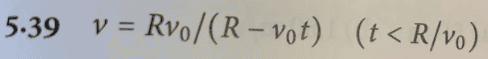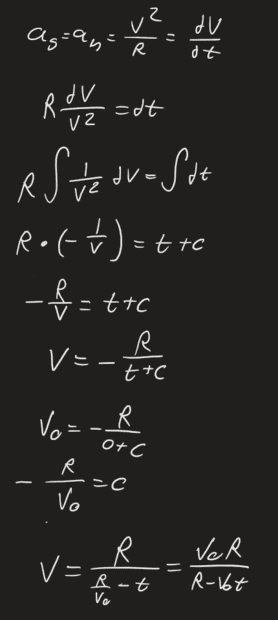# Circular Motion: Tangential and Normal Acceleration

• Heexit
In summary, the conversation on Physicsforum involved a user seeking help with a physics problem involving tangential acceleration and velocity. The conversation included a discussion on relevant equations and information needed to solve the problem. Ultimately, the user was able to come up with a solution with the help of others on the forum.

#### Heexit

Homework Statement
A particle moves in a circular path of radius R in such a way that the tangential acceleration is equal to the normal acceleration. Determine the velocity as a function of time t and the initial velocity (v_0).
Relevant Equations
N/A
Hello Physicsforum!

This is my attempt:
First I realised:
##a_s=a_n##

Secondly I used since previus known formulas:
##a_n=\frac {v^2} {R}##
##v=v_0+a_s*t##

Although now I do not know how to continue, any suggestions would be appriciated!
Thanks for your help on beforehandHeexit said:
##v=v_0+a_s*t##
That is only if the tangential acceleration has constant magnitude.
What equation relates ##a_s## to ##v_s## more generally?

Unless you have left out some information, there is no way to determine ##v_0##.

haruspex said:
That is only if the tangential acceleration has constant magnitude.
What equation relates ##a_s## to ##v_s## more generally?

Unless you have left out some information, there is no way to determine ##v_0##.

The only equation that I can think of is:
##a_s=\frac {dv} {dt}##
Anything more than that I can't think of :/

There might have been some inaccurate translation on my side, sorry about that. The question does not ask us for a define value of ##v_0##, rather an expression of variables, see solution in picture below:Heexit said:
The only equation that I can think of is:
##a_s=\frac {dv} {dt}##
That will do nicely. Combine that with your other information.
Heexit said:
The question does not ask us for a define value of ##v_0##, rather an expression of variables, see solution in picture below:
View attachment 327036
Ok.

•MatinSAR and Heexit••